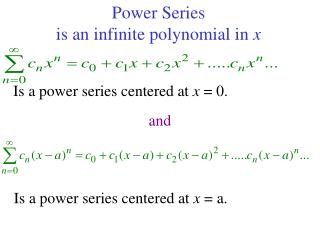# Power Series is an infinite polynomial in x - PowerPoint PPT PresentationDownload PresentationPower Series is an infinite polynomial in x

Power Series is an infinite polynomial in x
Download Presentation## Power Series is an infinite polynomial in x

- - - - - - - - - - - - - - - - - - - - - - - - - - - E N D - - - - - - - - - - - - - - - - - - - - - - - - - - -
##### Presentation Transcript

1. Power Series is an infinite polynomial in x Is a power series centered at x = 0. and Is a power series centered at x = a.

2. Examples of Power Series Is a power series centered at x = 0. and Is a power series centered at x = -1.

3. Geometric Power Series

4. The graph of f(x) = 1/(1-x) and four of its polynomial approximations .

5. Convergence of a Power Series There are three possibilities 1)There is a positive number R such that the series diverges for |x-a|> R but converges for |x-a|< R. The series may or may not converge at the endpoints, x = a - R and x = a + R. 2)The series converges for every x. (R = .) 3)The series converges at x = a and diverges elsewhere. (R = 0)

6. Review of tests for convergence 2. Using the ratio test: a) Series converges for L < 1 b) Series diverges for L >1 c) Test is inconclusive if L = 1 3. Using the root test: a) Series converges for L < 1 b) Series diverges for L >1 c) Test is inconclusive if L = 1 • Geometric Series • converges for | r | <1 • diverges for | r | >1 and | r | = 1

7. What is the interval of convergence? Since r = x, the series converges |x| <1, or -1 < x < 1. Test endpoints of –1 and 1. Series diverges Series diverges 1 -1 interval of convergence is (-1,1).

8. Geometric Power Series 1.Find the function 2. Find the radius of convergence R = 3

9. 3. Find the interval of convergence For x = -2, Test endpoints Geometric series with r < 1, converges For x = 4 By nth term test, the series diverges. Interval of convergence

10. Find interval of convergence Use the ratio test: 0 < 1 for all reals R=  Series converges for all reals. (-, ) Interval of convergence

11. Finding interval of convergence Use the ratio test: R=0 Series converges only for center point [0, 0] Interval of convergence

12. Finding interval of convergence Use the ratio test: (-1, 1) -1< x <1 R=1 Test endpoints For x = -1 For x = 1 Harmonic series diverges Alternating Harmonic series converges [-1, 1) Interval of convergence

13. Differentiation and Integration of Power Series If the function is given by the series Has a radius of convergence R > 0, on the interval (c-R, c+R) the function is continuous, differentiable and integrable where: and The radius of convergence is the same but the interval of convergence may differ at the endpoints.

14. Constructing Power Series If a power series exists has a radius of convergence = R It can be differentiated So the nth derivative is

15. Finding the coefficients for a Power Series All derivatives for f(x) must equal the series Derivatives at x = a.

16. If f has a series representation centered at x=a, the series must be If f has a series representation centered at x=0, the series must be

17. Form a Taylor Polynomial of order 3 for sin x at a =

18. The graph of f(x) = ex and its Taylor polynomials

19. Find the derivative and the integral

20. Taylor polynomials for f(x) = cos (x)